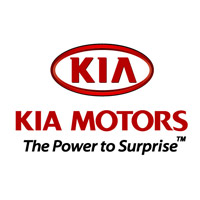# 狮跑本地参考价： 15.98 万元起 厂商指导价：15.98-19.68 万
 车市行情　｜　狮跑 降万
 最新年款： 2013款 级　别：紧凑型SUV 结　构：SUV 车身颜色： 红色 油　耗：6.8L/100km 排　量：2.0 内饰配色： 红黑 保　修：3年或6万公里 变速箱：MT|AT

#### 深港澳车展：起亚2013款狮跑正式发布#### 东风悦达起亚推出新狮跑 将于6月8日上市#### 2012款狮跑增配上市 售价保持不变2.0L 自然吸气 142马力 欧IV 官方指导价 本地参考价 车型信息 2013款 2.0L 手动两驱版GL 5挡手动 前置前驱 10.98万暂无 参数　图片　＋对比 2013款 2.0L 自动两驱版GL 4挡手自一体 前置前驱 11.98万暂无 参数　图片　＋对比 2013款 2.0L 手动两驱版GLS 5挡手动 前置前驱 12.38万暂无 参数　图片　＋对比 2013款 2.0L 自动两驱版GLS 4挡手自一体 前置前驱 13.38万暂无 参数　图片　＋对比 2013款 2.0L 手动四驱版GLS 5挡手动 前置四驱 13.88万暂无 参数　图片　＋对比 2013款 2.0L 自动两驱版DLX 4挡手自一体 前置前驱 13.68万暂无 参数　图片　＋对比 2013款 2.0L 自动两驱版Premium 4挡手自一体 前置前驱 14.68万暂无 参数　图片　＋对比

### 绕车实拍 外观 中控 座椅 细节 全部### 狮跑 新闻资讯#### 深港澳车展：起亚2013款狮跑正式发布

6月8日，东风悦达起亚在深港澳车展发布了2013款狮跑车型。据了解，新车型共计7款（比老款多出2.0LDLXAT车型），将于6月内上市销售，售价区间与...#### 东风悦达起亚推出新狮跑 将于6月8日上市#### 2012款狮跑增配上市 售价保持不变

2011年10月9日，东风悦达起亚2012款狮跑在全国范围内同步上市。此次改款涉及狮跑全系车型，升级后官方指导价格依然维持15.98万至19.68万元。#### 高性价比SUV车型推荐 合资品牌：新狮跑#### 东风悦达起亚新狮跑发布 售价或15日公布#### 变脸 试驾体验东风悦达起亚新狮跑#### 搅动自主品牌市场 新狮跑11.98万元起

SUV车市的又一轮激烈混战已经拉开序幕！日前，东风悦达起亚新狮跑直降4万，只要11.98万元起。也就是说，现在只要自主品牌的价格，就能买到优势...#### 搅动自主品牌市场 新狮跑11.98万元起

SUV车市的又一轮激烈混战已经拉开序幕！日前，东风悦达起亚新狮跑直降4万，只要11.98万元起。也就是说，现在只要自主品牌的价格，就能买到优势...#### 新乡诚盛与您聚“惠“日报社车展#### 搅动自主品牌市场 新狮跑11.98万元起

SUV车市的又一轮激烈混战已经拉开序幕！日前，东风悦达起亚新狮跑直降4万，只要11.98万元起。也就是说，现在只要自主品牌的价格，就能买到优势...#### 起亚全新狮跑11.98万元起

SUV车市的又一轮激烈混战已经拉开序幕！日前，东风悦达起亚新狮跑直降4万，只要11.98万元起。也就是说，现在只要自主品牌的价格，就能买到优势...#### “都市拓界车”新狮跑 全系直降4万元#### 河南万盛新狮跑直降4万元### 猜你喜欢

﻿
• 快速找车
• 选择品牌
• 选择品牌
• A  奥迪
• A  阿斯顿·马丁
• A  阿尔法·罗密欧
• B  宝沃
• B  布加迪
• B  巴博斯
• B  保时捷
• B  宾利
• B  奔驰
• B  宝马
• B  本田
• B  别克
• B  标致
• B  比亚迪
• B  宝骏
• B  北汽制造
• B  北汽新能源
• B  北汽幻速
• B  北汽威旺
• B  北京汽车
• B  奔腾
• B  北汽绅宝
• B  北汽昌河
• C  长安欧尚
• C  长安
• C  长安凯程
• C  长城
• D  大众
• D  道奇
• D  DS
• D  东南
• D  东风风神
• D  东风风行
• D  东风小康
• D  东风风度
• D  东风
• F  福特
• F  丰田
• F  菲亚特
• F  法拉利
• F  福田
• F  福迪
• F  福汽启腾
• G  观致
• G  广汽传祺
• G  广汽吉奥
• G  GMC
• H  红旗
• H  汉腾汽车
• H  哈弗
• H  哈飞
• H  海格
• H  海马
• H  华颂
• H  黄海
• H  华泰
• H  恒天
• J  捷途
• J  几何汽车
• J  捷达
• J  吉利汽车
• J  捷豹
• J  Jeep
• J  江淮
• J  江铃
• J  金杯
• J  九龙
• J  金旅
• K  凯翼
• K  凯迪拉克
• K  克莱斯勒
• K  科尼塞克
• K  卡威
• K  开瑞
• L  路虎
• L  林肯
• L  劳斯莱斯
• L  兰博基尼
• L  雷克萨斯
• L  铃木
• L  领克
• L  雷诺
• L  理念
• L  力帆
• L  莲花汽车
• L  猎豹
• L  路特斯
• L  陆风
• M  马自达
• M  MG
• M  MINI
• M  玛莎拉蒂
• M  摩根
• M  迈凯轮
• N  纳智捷
• O  欧拉
• O  欧宝
• O  讴歌
• O  欧朗
• Q  奇瑞
• Q  起亚
• Q  启辰
• R  日产
• R  荣威
• R  瑞麒
• S  SERES赛力斯
• S  三菱
• S  斯威汽车
• S  萨博
• S  smart
• S  斯柯达
• S  斯巴鲁
• S  思铭
• S  双龙
• S  上汽大通
• S  双环
• T  特斯拉
• T  腾势
• W  蔚来
• W  沃尔沃
• W  WEY
• W  五菱汽车
• W  五十铃
• W  威兹曼
• W  威麟
• X  现代
• X  雪佛兰
• X  星途
• X  雪铁龙
• X  小鹏汽车
• X  西雅特
• Y  一汽
• Y  英菲尼迪
• Y  英致
• Y  依维柯
• Y  野马汽车
• Y  永源
• Z  众泰
• Z  中华
• Z  中兴
• Z  知豆
• 选择车系
• 选择车系
• 车型对比
• 选择品牌
• 选择品牌
• A  奥迪
• A  阿斯顿·马丁
• A  阿尔法·罗密欧
• B  宝沃
• B  布加迪
• B  巴博斯
• B  保时捷
• B  宾利
• B  奔驰
• B  宝马
• B  本田
• B  别克
• B  标致
• B  比亚迪
• B  宝骏
• B  北汽制造
• B  北汽新能源
• B  北汽幻速
• B  北汽威旺
• B  北京汽车
• B  奔腾
• B  北汽绅宝
• B  北汽昌河
• C  长安欧尚
• C  长安
• C  长安凯程
• C  长城
• D  大众
• D  道奇
• D  DS
• D  东南
• D  东风风神
• D  东风风行
• D  东风小康
• D  东风风度
• D  东风
• F  福特
• F  丰田
• F  菲亚特
• F  法拉利
• F  福田
• F  福迪
• F  福汽启腾
• G  观致
• G  广汽传祺
• G  广汽吉奥
• G  GMC
• H  红旗
• H  汉腾汽车
• H  哈弗
• H  哈飞
• H  海格
• H  海马
• H  华颂
• H  黄海
• H  华泰
• H  恒天
• J  捷途
• J  几何汽车
• J  捷达
• J  吉利汽车
• J  捷豹
• J  Jeep
• J  江淮
• J  江铃
• J  金杯
• J  九龙
• J  金旅
• K  凯翼
• K  凯迪拉克
• K  克莱斯勒
• K  科尼塞克
• K  卡威
• K  开瑞
• L  路虎
• L  林肯
• L  劳斯莱斯
• L  兰博基尼
• L  雷克萨斯
• L  铃木
• L  领克
• L  雷诺
• L  理念
• L  力帆
• L  莲花汽车
• L  猎豹
• L  路特斯
• L  陆风
• M  马自达
• M  MG
• M  MINI
• M  玛莎拉蒂
• M  摩根
• M  迈凯轮
• N  纳智捷
• O  欧拉
• O  欧宝
• O  讴歌
• O  欧朗
• Q  奇瑞
• Q  起亚
• Q  启辰
• R  日产
• R  荣威
• R  瑞麒
• S  SERES赛力斯
• S  三菱
• S  斯威汽车
• S  萨博
• S  smart
• S  斯柯达
• S  斯巴鲁
• S  思铭
• S  双龙
• S  上汽大通
• S  双环
• T  特斯拉
• T  腾势
• W  蔚来
• W  沃尔沃
• W  WEY
• W  五菱汽车
• W  五十铃
• W  威兹曼
• W  威麟
• X  现代
• X  雪佛兰
• X  星途
• X  雪铁龙
• X  小鹏汽车
• X  西雅特
• Y  一汽
• Y  英菲尼迪
• Y  英致
• Y  依维柯
• Y  野马汽车
• Y  永源
• Z  众泰
• Z  中华
• Z  中兴
• Z  知豆
• 选择车系
• 选择车系
• 选择车型
• 选择车型
• 意见反馈